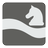The Shortest Knight's Path
Moderate
English RU

Sofi found a chess set in the supply closet on the robots ship. She has decided to learn how to play the game of chess starts by attempting to understand how the knight moves.

Chess is played on a square board of eight rows (called ranks and denoted with numbers 1 to 8) and eight columns (called files and denoted with letters a to h) of squares.

The knight moves to any of the closest squares that are not on the same rank, file, or diagonal, thus the move forms an "L"-shape: two squares vertically and one square horizontally, or two squares horizontally and one square vertically.

You are given the start and end squares as chess coordinates separated by a hyphen. You should find the length of the shortest path for the knight from one point to another on the chessboard.Input: Squares coordinates as a string.

Output: The number of moves in the shortest path the knight can take as an integer.

Example:

```checkio("b1-d5") == 2, "First"
checkio("a6-b8") == 1, "Second"
checkio("h1-g2") == 4, "Third"
checkio("h8-d7") == 3, "Fourth"
checkio("a1-h8") == 6, "Fifth"
```

How it is used: Let's think about chess and how we can use pathfinding and computer science to play this awesome game. The game structure and nature of chess is related to several branches of mathematics. Many combinatorial and topological problems connected to chess have been known for hundreds of years.

Precondition: An input string satisfies regexp "[a-h][1-8]-[a-h][1-8]".
start_cell != end_cell